# Franklin system

(diff) ← Older revision | Latest revision (diff) | Newer revision → (diff)

One of the classical orthonormal systems of continuous functions. The Franklin system(see  or ) is obtained by applying the Schmidt orthogonalization process (cf. Orthogonalization method) on the interval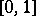to the Faber–Schauder system, which is constructed using the set of all dyadic rational points in; in this case the Faber–Schauder system is, up to constant multiples, the same as the system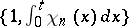, where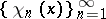is the Haar system. The Franklin system was historically the first example of a basis in the space of continuous functions that had the property of orthogonality. This system is also a basis in all the spaces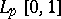,(see ). If a continuous functiononhas modulus of continuity, andis the partial sum of orderof the Fourier series ofwith respect to the Franklin system, then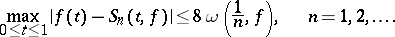Here the Fourier–Franklin coefficients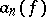ofsatisfy the inequalitiesand the conditions

a),;

b),;

c)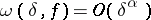,; are equivalent for.

If the continuous functionis such that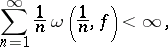then the seriesconverges uniformly on, and if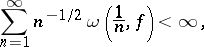then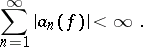All these properties of the Franklin system are proved by using the inequalities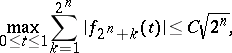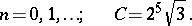The Franklin system is an unconditional basis in all the spaces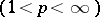and, moreover, in all reflexive Orlicz spaces (see ). Ifbelongs to,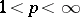, then one has the inequality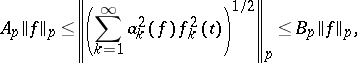wheredenotes the norm in, and the constants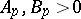depend only on.

The Franklin system has had important applications in various questions in analysis. In particular, bases in the spaces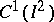(see ) and(see ) have been constructed using this system. Hereis the space of all continuously-differentiable functionson the square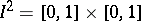with the normand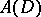, the disc space, is the space of all functionsthat are analytic in the open disc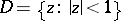in the complex plane and continuous in the closed disc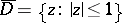with the norm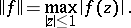The questions of whether there are bases inandwere posed by S. Banach .

How to Cite This Entry:
Franklin system. Encyclopedia of Mathematics. URL: http://encyclopediaofmath.org/index.php?title=Franklin_system&oldid=16655
This article was adapted from an original article by B.I. Golubov (originator), which appeared in Encyclopedia of Mathematics - ISBN 1402006098. See original article